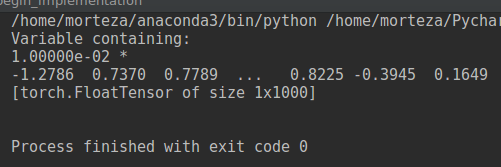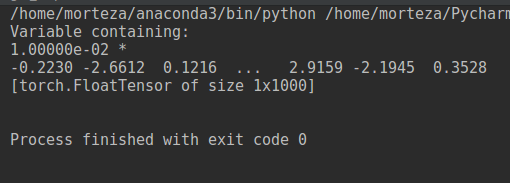# Loading a saved model in two ways and getting different results

Hi
I loaded a a saved model in two ways. But when I feed an image, the results of softmax layer are not the same. I don’t know what’s the reason. My piece of codes are as follow.

my first method is:

``````  class myModel(nn.Module):
def __init__(self):
super(myModel,self).__init__()
self.vgg_model.eval()
self.convs=[]
for child in self.vgg_model.children():
self.convs.append(child)

def forward(self,x):
for i in range(9):
x = self.convs[i](x)
x = x.view(-1, 1 * 1 * 512)
x = self.convs(x)
print(x)
return x
``````

the second method is:

``````mymodel = torch.loard('bestmodel.pt')
``````

I used the transfer learning on resnet for ants and bees dataset. The class prediction of the network for input images are similar, but the returned real values in softmax are not the same. As a matter of fact, I am worry about bad effects of this event in my future works.

Please guide me what is the reason for this difference?
does the first method uses weights of the saved model? or does it only use the definition of different kind of layers and randomly initialize the weights?

you are assuming that

`````` for child in self.vgg_model.children():
self.convs.append(child)
``````

will return layers in some kind of sequential order, that might or might not hold true. maybe that wrong assumption is the issue in your case.

As a matter of fact I loaded resnet model and use the name of vgg_model incorrectly. There is no error and all things work fine without error. But I check your suggestion. Let me check and reply again

Hi
I checked and faced with an event in VGG network that was odd.
This is a sample code of using VGG16:

``````trained_model = torchvision.models.vgg16() #myModel()  #
trained_model.eval()
############ Import new image for classification #########################
img.show()
img = Variable(img)
img = img.unsqueeze(0)
pred = trained_model(img)
print(pred)
``````

Now see outputs for each run:
First run:The second run:I feed one image in each run but the results are not the same. It is odd because I think all weights are constant after training and there is no change. Therefore, when we feed constant input image, we must get the same result values in the softmax layer without any change. However, it is not true here. Can you tell me what is the problem?